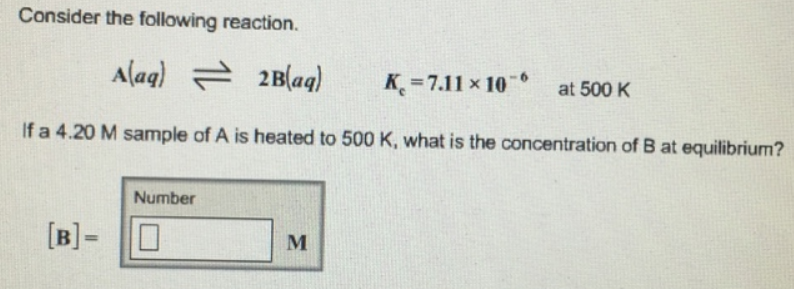# Problem: Consider the following reaction. A (aq) ⇌ 2B (aq)               K  c = 7.11 x 10 -6 at 500 Klf a 4.20 M sample of A is heated to 500 K, what is the concentration of B at equilibrium?

###### FREE Expert Solution
89% (472 ratings)###### Problem Details

Consider the following reaction.

A (aq) ⇌ 2B (aq)               K  c = 7.11 x 10 -6 at 500 K

lf a 4.20 M sample of A is heated to 500 K, what is the concentration of B at equilibrium?